### Home > PC > Chapter 1 > Lesson 1.2.1 > Problem1-67

1-67.
1. Find an equation for each graph below. State the parent graph and describe the transformation. Homework Help ✎

1.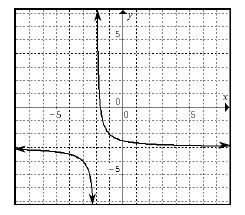2.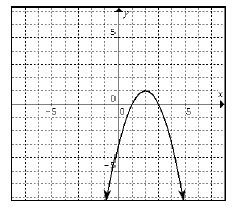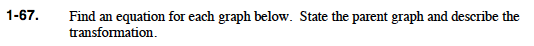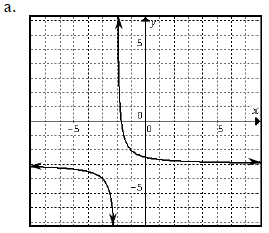What is the parent graph?

Rewrite the parent graph reflecting the horizontal shift.

Rewrite the parent graph again to reflect the vertical shift.

$y= \frac{1}{x}$

$y = \frac{1}{x+2}$

$y=\frac{1}{\textit{x} + 2} - 3$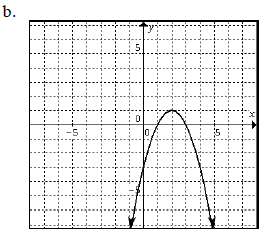Follow the same procedure used for (a).